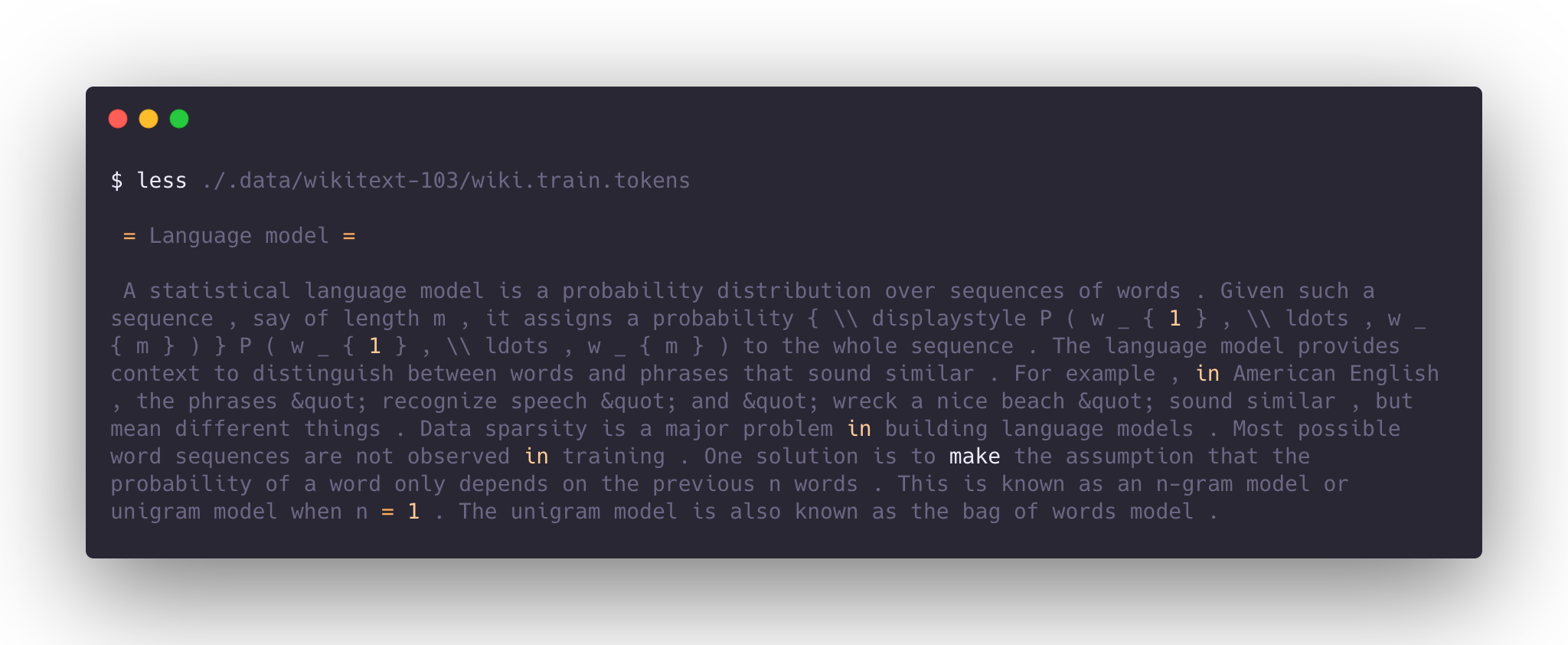# WikiText-103You can view the WikiText-103 leaderboard here.

## Getting Started

You'll need the following in the root of your repository:

• `sotabench.py` file - contains benchmarking logic; the server will run this on each commit
• `requirements.txt` file - Python dependencies to be installed before running `sotabench.py`
• `sotabench_setup.sh` (optional) - any advanced dependencies or setup, e.g. compilation

You can write whatever you want in your `sotabench.py` file to get language model predictions on the WikiText-103 dataset.

But you will need to record your results for the server, and you'll want to avoid doing things like downloading the dataset on the server. So you should:

• Point to the server WikiText-103 data path - popular datasets are pre-downloaded on the server.
• Include an Evaluation object in `sotabench.py` file to record the results.
• Use Caching (optional) - to speed up evaluation by hashing the first batch of predictions.

We explain how to do these various steps below.

## Server Data Location

The WikiText-103 development data is located in the root of your repository on the server at `.data/nlp/wikitext-103/wikitext-103-v1.zip`. The archive contains a folder `wikitext-103` with the following files:

• `wiki.train.tokens`
• `wiki.valid.tokens`
• `wiki.test.tokens`

It is the original zip file released here. We are running the benchmark on the `wiki.test.tokens` dataset. We have two helper methods that will unpack the dataset for you and give you the `pathlib.Path` to the test file.

The first option `test_set_path` is available once you instantiate the `WikiText103Evaluator`:

```...

evaluator = WikiText103Evaluator(
model_name="Transformer-XL Large",
paper_arxiv_id="1901.02860",
paper_pwc_id="transformer-xl-attentive-language-models",
local_root='/content/wikitext-103'
)
# dataset_path is pathlib.Path and points to wikitext.test.tokens
with evaluator.test_set_path.open() as f:
```

There is a second option available if you are evaluating multiple models and need to use the same dataset multiple times - `WikiText103Evaluator.get_test_set_path(local_root)`. This will get the path before you initialize a WikiText evaluator:

```from sotabencheval.language_modelling import WikiText103Evaluator

test_file_path = WikiText103Evaluator.get_test_set_path('/home/ubuntu/my_data/wiki103')
with test_file_path.open() as f:
```

## How Do I Initialize an Evaluator?

Add this to your code - before you start batching over the dataset and making predictions:

```from sotabencheval.language_modelling import WikiText103Evaluator

evaluator = WikiText103Evaluator(model_name='Model name as found in paperswithcode website')
```

If you are reproducing a model from a paper, then you can enter the arXiv ID. If you put in the same model name string as on the Wikitext-103 leaderboard then you will enable direct comparison with the paper's model. If the `arxiv_id` is not available you can use `paperswithcode.com` id. Below is an example of an evaluator that matches `Transformer XL`:

```from sotabencheval.language_modelling import WikiText103Evaluator

evaluator = WikiText103Evaluator(
model_name="Transformer-XL Large",
paper_arxiv_id="1901.02860",
paper_pwc_id="transformer-xl-attentive-language-models",
local_root="path_to_your_data",
)
```

The above will directly compare with the result of the paper when run on the server.

## How Do I Evaluate Predictions?

The evaluator object has an `.add(log_probs, targets)` method to submit predictions by batch or in full. We expect you to give us the log probability of a batch of target tokens and the `target` tokens themselves. The `log_probs` can be either:

• a 0d "tensor" (`np.ndarray`/`torch.tensor`) - summed log probability of all `targets` tokens
• a 2d "tensor" (`np.ndarray`/`torch.tensor`) - log probabilities of each target token, the `log_probs.shape` should match `targets.shape`
• a 3d "tensor" (`np.ndarray`/`torch.tensor`) - distribution of log probabilities for each position in the sequence, we will gather the probabilities of target tokens for you.

It is recommended to use third or second option as it allows us to check your perplexity calculations.

If your model uses subword tokenization you don't need convert subwords to full words. You are free to report probability of each subword: we will adjust the perplexity normalization accordingly. Just make sure to set `subword_tokenization=True` in your evaluator.

Here is an example of how to report results (for a PyTorch example):

```evaluator = WikiText103Evaluator(
model_name='GPT-2 Small',
local_root="path_to_your_data",
subword_tokenization = True
)

# run you data preprocessing, in case of GPT-2 the preprocessing removes moses artifacts
model.eval()
output = model(input)
log_probs = torch.LogSoftmax(output, dim=-1)
target_log_probs = output.gather(-1, targets.unsqueeze(-1))
```

When you are done, you can get the results locally by running:

```evaluator.get_results()
```

But for the server you want to save the results by running:

```evaluator.save()
```

This method serialises the results and model metadata and stores to the server database.

## How Do I Cache Evaluation?

Sotabench reruns your script on every commit. This is good because it acts like continuous integration in checking for bugs and changes, but can be annoying if the model hasn't changed and evaluation is lengthy.

Fortunately sotabencheval has caching logic that you can use.

The idea is that after the first batch, we hash the model outputs and the current metrics and this tells us if the model is the same given the dataset. You can include hashing within an evaluation loop like follows (in the following example for a PyTorch repository):

```with torch.no_grad():
# ...
output = model(input)
log_probs = #...

if evaluator.cache_exists:
break

evaluator.save()
```

If the hash is the same as in the server, we infer that the model hasn't changed, so we simply return hashed results rather than running the whole evaluation again.

Caching is very useful if you have large models, or a repository that is evaluating multiple models, as it speeds up evaluation significantly.

## A full sotabench.py example

Below we show an implementation for a model from the `huggingface/transformers`. This incorporates all the features explained above: (a) using the server data, (b) using the WikiText-103 Evaluator, and (c) caching the evaluation logic:

```import torch
from tqdm import tqdm
from sotabencheval.language_modelling import WikiText103Evaluator

evaluator = WikiText103Evaluator(
model_name="Transformer-XL Large",
paper_arxiv_id="1901.02860",
paper_pwc_id="transformer-xl-attentive-language-models",
local_root='/content/wikitext-103'
)

with evaluator.test_set_path.open() as f:

seq_len = 128
evaluator.reset_timer()
model.eval()
X, Y, mems = test_data[None, :-1], test_data[None, 1:], None
for s in tqdm(range(0, X.shape[-1], seq_len)):
x,y = X[..., s:s+seq_len].to("cuda"), Y[..., s:s+seq_len].to("cuda")
log_probs, mems, *_ = model(input_ids=x, mems=mems)